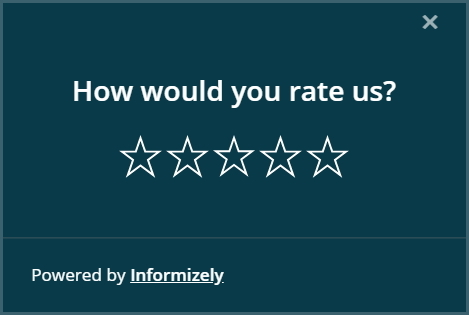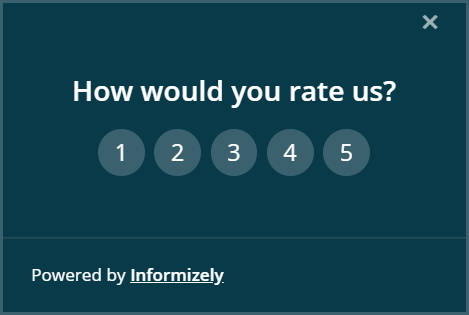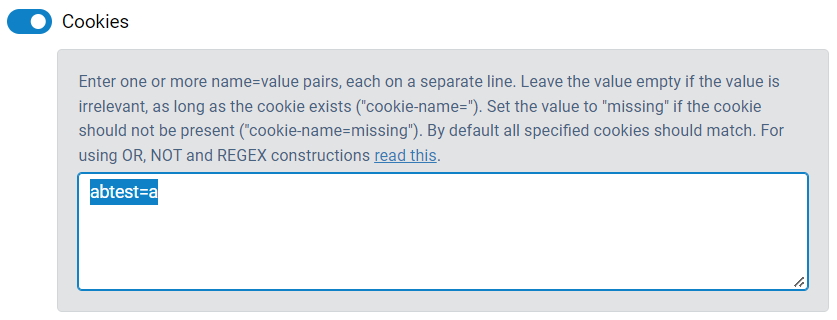# Optimize your website surveys with A/B Testing

In some cases you may wonder if a specific survey can be improved upon to get a higher response rate or higher quality responses, for example by varying:

• The opening text
• The color scheme
• The position on the screen
• The in-animation
• The trigger used to show the survey, e.g. time on page versus scroll percentage
• Ask multiple questions on one page, or each question on a separate page
Or by changing something else.

#### An example

It's pretty simple to A/B test 2 or more surveys. As an example we're going to show how to test 2 variations of a short survey asking the simple question "How would you rate us?", where one survey (the "A" survey) asks to select between 1 and 5 stars, and the other survey (the "B" survey) asks to select a number between 1 and 5.To implement A/B testing in this example we use cookie targeting and a little bit of JavaScript.
First we create survey A and fully configure it. On the "TARGET > ADVANCED" tab, section we configure the survey to be shown only when the cookie "abtest" has the value "a" (the name of the cookie can be anything, we use "abtest" here):Then we publish the survey and we create a copy by clicking the "More" icon and then the "Copy" icon in the survey panel. We then make the necessary changes to the copy (the "B" survey), in this case changing the "stars" answer to a "numbers" answer. In the cookie targeting setting we configure this survey to be shown only when the the cookie "abtest" has the value "b". Then we publish the survey.

Now our "A" and "B" surveys are both published, but neither will be shown because the cookie "abtest" is never set. For this we need a little bit of JavaScript to be added to the website page(s) where these surveys can be shown. The following JavaScript should be added before the Informizely code snippet that loads your Site's survey configuration:

```<script type="text/javascript">
setCookie("abtest", (Math.random() < 0.5) ? "a" : "b", 365);
</script>
```

For this example we asign equal weights to the "A" and "B" survey, but it's easy to change this if needed. Changing "0.5" to "0.8" for example will show the "A" survey to 80% of all visitors and the "B" survey to the other 20%.

The code above uses the JavaScript functions 'getCookie' and 'setCookie' that may already be present on the page. If not, you can add them before the code above, as follows:

```<script type="text/javascript">
{
var name = cname + "=";
for (var i = 0; i < ca.length; i++) {
var c = ca[i];
while (c.charAt(0) == ' ')
c = c.substring(1);
if (c.indexOf(name) == 0)
return c.substring(name.length, c.length);
}
return "";
}